﻿ Lamb Shift (Hydrogen atom)

# Lamb shift is an illusion ?

Youhei Tsubono

## Dirac's hydrogen = Bohr-Sommerfeld model.

### [ What if Dirac's hydrogen is wrong ? ]

(Fig.1) Dirac's hydrogen = Bohr-Sommerfeld model.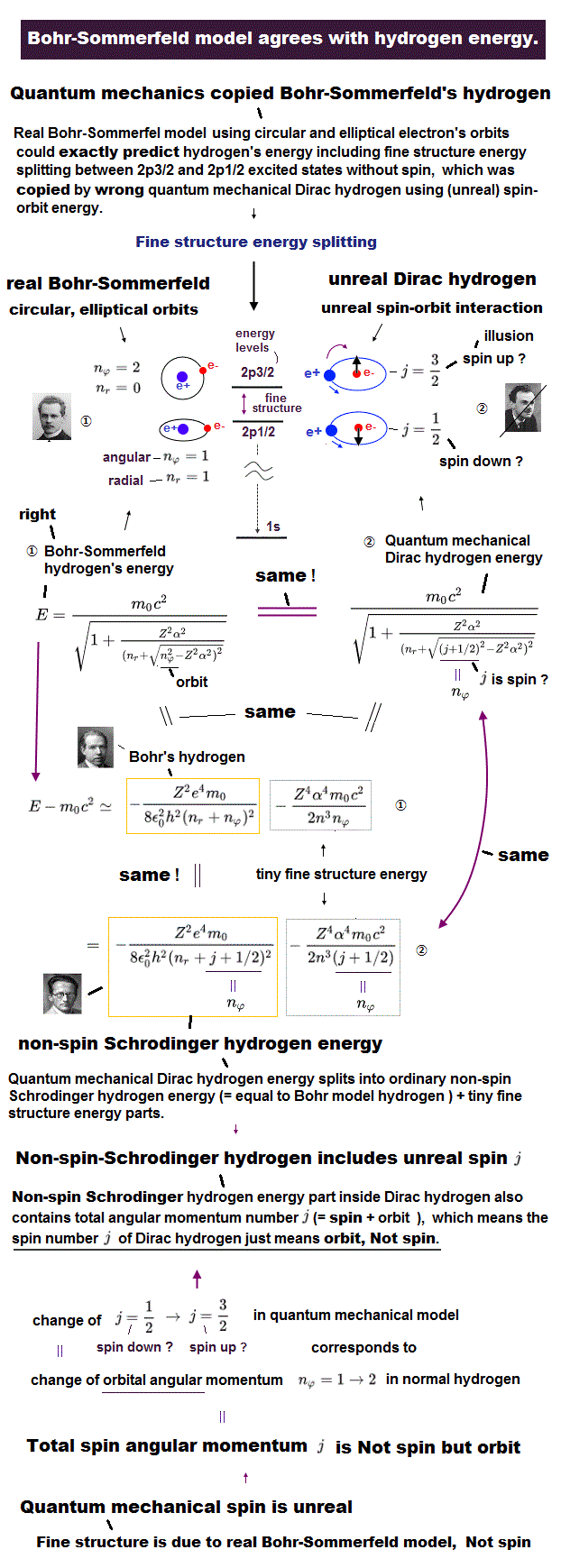As shown in this page, fine structure was first explained by Bohr-Sommerfeld model in 1916.
And Later Dirac's hydrogen accidentally got the same solutions as Bohr-Sommerfeld model.

Though Dirac's hydrogen has spin, observed spectrum lines of hydrogen atom are much fewer than expected by Dirac's hydrogen.
They insist, many accidental coincidences ( 2s1/2 = 2p1/2, ... ) happen, so observed lines appears to be fewer.

(Fig.2) Dirac's hydrogen includes many "fictional" states.The problems is Dirac's hydrogen has many wrong states such as 1p1/2 and 2d3/2 ... as shown in this page.
If these wrong states do not exist, Dirac's hydrogen cannot get the solutions.
For example, 1p1/2 state is the energy level n = 1 and the orbital angular momentum l = 1, which does NOT exist in quantum mechanical hydrogen.
This clearly shows Dirac's hydrogen is wrong, and Bohr-Sommerfeld model is right.

(Fig.3) Right angle lever rotates or not ?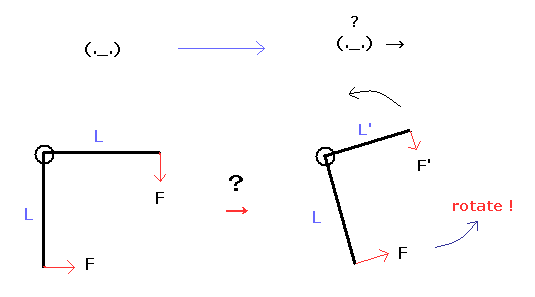Furthermore, various fatal paradoxes clearly shows special relativity is wrong.
Dirac equation is first-order in energy and momentum, and includes 4×4 γ matrices.
These forms of Dirac equations are just equal to Klein-Gordon equation (= special relativity ).
On the other hand, Bohr-Sommerfeld model does NOT use peculiar form to relativity such as E2/c2 = p2 + (mc)2, but use usual relation of first-order energy = T + V.

Of course, if special relativity is wrong, Dirac equation relying on γ matrices is also wrong.
So we need to reconsider the interpretation of Lamb shift.
Because this very tiny Lamb shift completely depends on the assumption that Dirac's hydrogen is right.

## We cannot confirm what Lamb shift really means.

### [ Lamb shift is too small. ]

(Fig.3) Lamb shift is real ?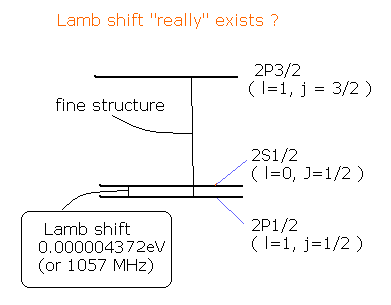Dirac's hydrogen gives the same energy values in 2s1/2 and 2p1/2.
And the quantum electrodynamics, which artificially removes infinity, is said to give very small energy difference between them.
This is called Lamb shift, which value is about one-tenth of fine structure and almost same as nuclear hyperfine structure.

Of course, this very small value (= 0.0000043 eV ) cannot be observed directly in the usual spectrum lines.
They just estimate this existence under the assumption that Dirac's hydrogen is right.
The important point is that we have NO ways to confirm Lamb shift really means the energy difference between 2s1/2 and 2p1/2.

(Fig.4) Electron collision method.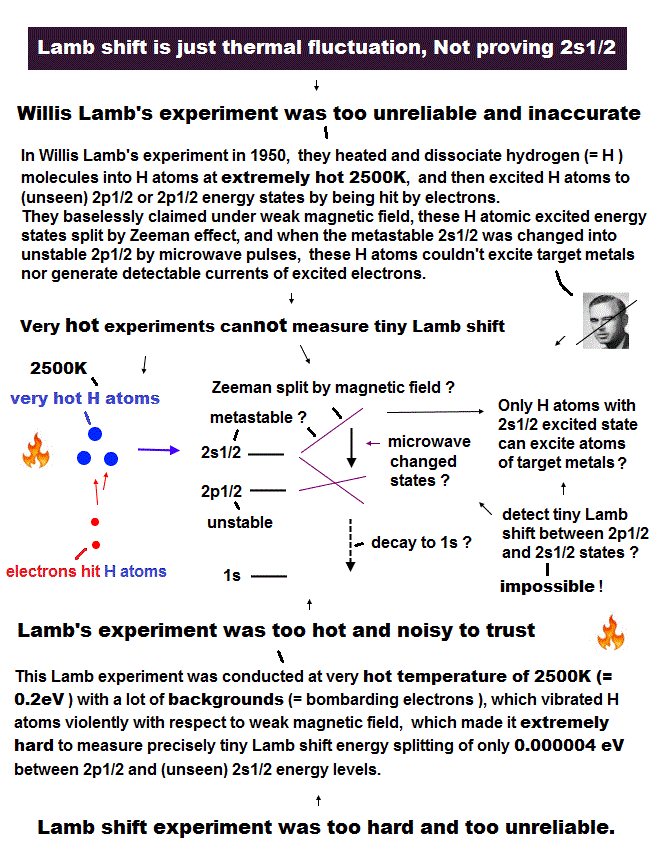Original expriment confirming Lamb shift used the mothods such as anomalous Zeeman effect, resonance and collision. 
As shown in Fig.4, excited hydrogen atom is exposed to electromagnetic radiation of some frequency under the magnetic field.
Under the very weak magnetic field, hydrogen atom is said to show anomalous Zeeman effect.
But this is only speculation, Because it is famous that hydrogen atom gives much fewer spectrum lines than sodium, and usually shows normal Zeeman effect.
( One of Balmer lines show even-split lines, but this lines are fewer than sodium, and can be expected by Bohr-Sommerfeld fine structure. )

(Fig.5) Fine-structure = "photon" spin 1 ??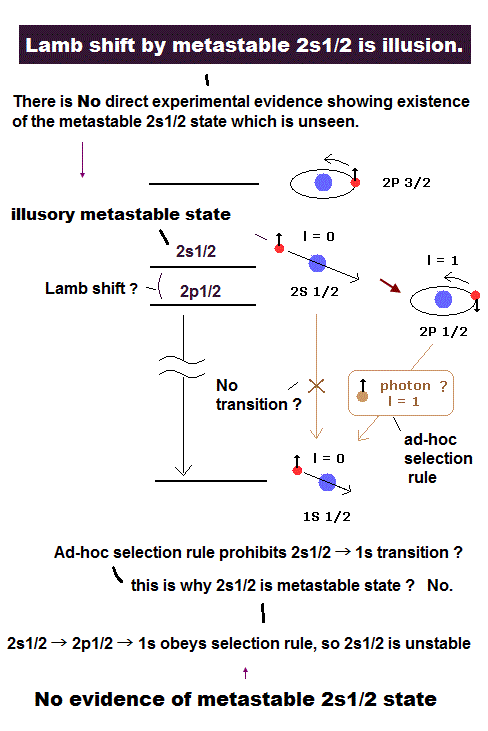It is said 2s1/2 is more stable than 2p1/2 due to the selection rule.
This selection rule is said to be caused by photon spin "1".
So, this metastable 2s1/2 state hydrogen can reach tungsten foil of Fig.4, and excite electron and produces electric current, they insist.
When the electromagnetic radiation induces transitions from 2s/12 to 2p1/2, which soon decays into the ground state and doesn't excite the foil.
So by measuring the light frequency needed for this transition, we can know the Lamb shift ?

The important point is that we can NOT know "metastable" state really means 2s1/2.
Furthermore, later Willis Lamb himself said "there is no such thing as a photon.", which means metastable 2s1/2 is wrong.

(Fig.6) Delayed choice experiment = Many-worlds = photon.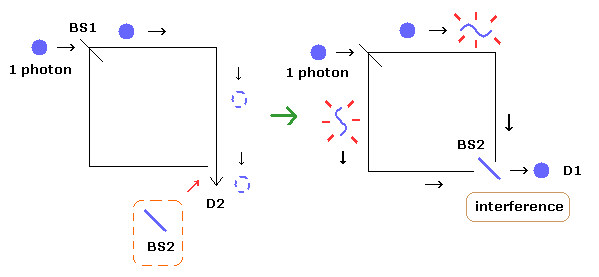As shown in this page, the existence of strange photon causes unrealistic many-worlds and faster-than light spooky link.

### [ Reconsideration of Lamb's original paper.]

According to Lamb's original paer , there are various opinions about the metasbale 2S1/2 state.
For example, Franck and Jordan gave reasons for doubting that the 2S1/2 level would be metastable .
And Snoek, and von Keussler investigated the relative intensities of H line and concluded that the 2S1/2 level was not appreciably metastable .
(Of course, there were some people who supported the metastability of 2S1/2 state.)
In this situation, Lamb tried to examine the Lamb shift assuming that the 2S1/2 was metastable.
(In the singly ionized helium (He+), he could not get the metastable state.)

### [ Anomalous Zeeman effect in fictitious Lamb shift disobeys Lande g factor, so spin is unreal. ]

(Fig.7) Anomalous Zeeman effect should be symmetrical, but ↓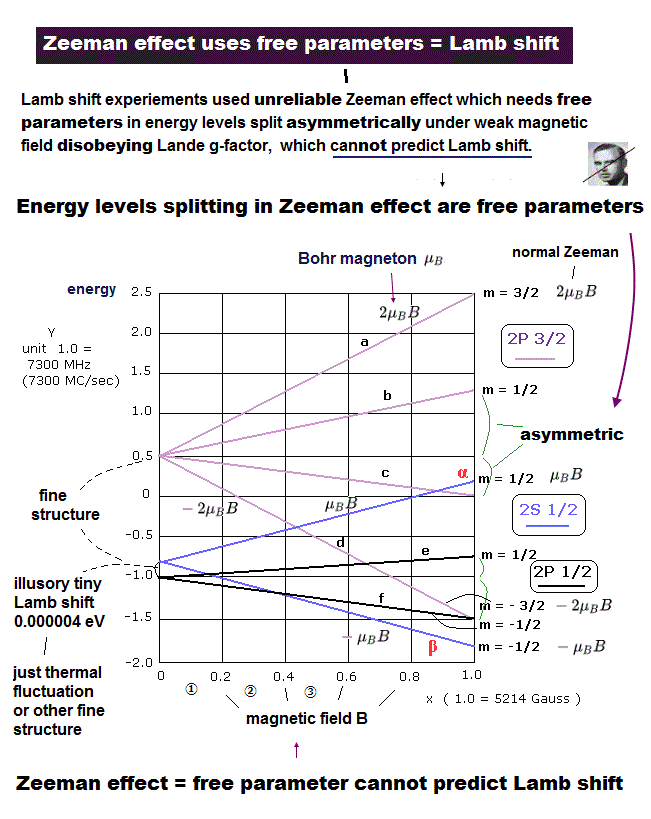A hydrogen atom with only one electron is known to generate normal Zeeman effect (= hence, there is No proof of electron spin ), and the anomalous Zeeman effect allegedly caused by (unreal) spin of the hydrogen can Not be observed in the ordinary spectral lines, except the physicists baselessly claim it might relate to the unseen (imaginary) tiny,tiny Lamb shift energy splitting.

Assuming anomalous Zeeman effect is always correct even in hydrogen atom, the energy level of the 2P3/2 electron splits into the following four levels according to Lande g factor ( this p.2 ).

μBH × 4/3 × 3/2 = BH ( m=3/2 )
μBH × 4/3 × 1/2 = 2/3 μBH ( m=1/2 )
μBH × 4/3 × -1/2 = -2/3 μBH ( m= -1/2 )
μBH × 4/3 × -3/2 = -2μBH ( m= -3/2 )

If Lande-g-factor rule is satisfied, each energy line has to split symmetrically around the original line.  But this doesn't happen, so spin is unreal.

As shown in Fig.7, they insist m = 1/2 and m = -1/2 states don't satisfy this Lande g factor.
If they satisfy Lande g factor, the energy level splits into upper and lower portions "symmetrically" (= ± 2/3 μBH ).
But in Fig.7, these are "asymmetric" ( this p.5 ).
This means that Lange g factor is NOT valid in the states of m = ± 1/2.

They claimed that m = ± 1/2 states needed to satisfy the very complicated equation of
(Eq.1)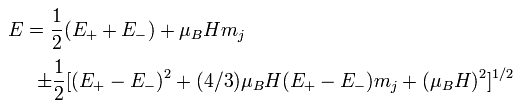where E+ and E- are zero field energies of 2P3/2and 2P1/2.
But of course, we can not know that this complicated equation is really valid also in the very weak magnetic field in the hydrogen atom.
(If this equation is not valid, the interpretation of Lamb shift would change completely.)

Also in the 2P1/2 state ( j=1/2, l=1, s=1/2, m=±1/2 ), the following Lande g factor is NOT valid, as shown in Fig.7.
( Remember that these definitions are only speculations, because these lines cannot be directly observed in the usual spectrum lines. )

μBH × 2/3 × 1/2 = 1/3 μBH ( m=1/2 )
μBH × 2/3 × -1/2 = -1/3 μBH ( m= -1/2 )

(Fig.8) Observed transitions ? (small circles).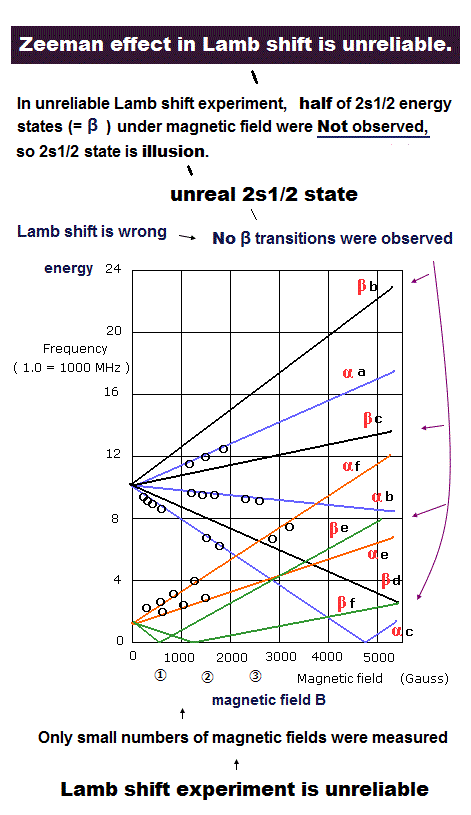Fig.8 data is from this,  this p.18,  this p.4.

As I said above, if transitions between 2s1/2 and 2p1/2 states happen, foil currents in Fig.4 may change.
From Fig.7, the resonance electromagnetic wave frequencies needed for the transitions can be estimated like Fig.8.
(For example, "α a" line means the microwave frequencies needed for the transition from 2S1/2 "α" state into 2P3/2 "a" state in the various magnetic fields. )
They claimed that the small circles were observed frequencies, so the Lamb shift of about 1000 MHz could be gotten.
But actually, it was very difficult to get the precise results in this method.

First, the hyperfine structure by the nuclear spin can not be ignored, because Lamb shift is very small.
So they needed to remove these effects "theoretically" from the observed wide spectrum linewidth.
They claimed that when the transitions from 2S into 2P1/2 or 2P3/2 occurred, it became unstable and falled into the 1S state.
Then the hydrogen atoms bump into the detection plates (= foil in Fig.4 ).
They supposed that the 1S state hydrogen could not excite the electrons, and 2S state could excite them, which were observed as electric current.
By observing the ejected electric current from the plates, they judged whether the transitions (2S → 2P → 1S) occurred or not.
Due to the wide line width and the heat noises, they could not precisely determine the resonance points.
(So the small circle points of Fig.8 could not be determined precicely.)

And as shown in Fig.8, the β state of 2S1/2 in Fig.7 was NOT observed (= no circle ), though it was also metastable state.
This is very strange.
And the distribution of the small circles in Fig.8 is "irregular".
So I wonder why other points of the lines in Fig.8 were not observed.

### [ Saturation Spectroscopy. ]

(Fig.9) Saturation Spectroscopy.Hydrogen collision methods are rough and not proper to confirm very small value of Lamb shift.
Doppler-free saturation spectroscopy passes the light with very narrow linewidth through a collection of hydrogen atoms undergoing Doppler-broadened transitions in a discharge tube.

But as I said, Lamb shift is as small as hyperfine structure of nuclear spin, so we cannot know this resonance really mean Lamb shift.
Some slight vibrations of nucleus and electrons may influence these results.
And some resonances such as 3s1/2-2p3/2 are hard to detect, and pseudoresonances (= cross resonance ) are mixed.

In Bohr-Smmerfeld model, some jagged signals ( 2s1/2, 2p1/2 are involved ) of Fig.9 right show elliptic orbit, which is closer to the nucleus.
So the right jagged waves may be related to the nuclear motion.

### [ If Dirac's hydrogen is wrong, we must change its interpretation. ]

The important point is that we have no ways to confirm these very small values really mean Lamb shift.
These interpretaions are completely dependent on the assumption that Dirac's hydrogen is right.

(Fig.10) Dirac's hydrogen = Non relativistic.As shown in this page, Hamiltonian of Dirac hydrogen is not relativistic covariant form.
Because Coulomb potential energy transforms complicatedly under Lorentz transformation like Fig.10.
This means the true relativistic Dirac's hydrogen ( if it exists ) gives different energy solution.

Lamb shift is very tiny, so if this form of Coulomb potential changes under the proton or electron's slight motion, its value changes.
So the interpretation of Lamb shift needs to be changed.

## Lamb shift is explained by Sommerfeld fine structure.

(Fig.11)  Artificial selection rule "limits" transitions.Bohr-Sommerfeld model gives exact spectrum lines (= fine structure ), which accidentally agreed to quantum mechanics later !

Its agreement is amazing, because Bohr model doesn't have unrealistic spin, and quantum mechanics does.  They say energy levels of 2s1/2 and 2p1/2 are the same.

Later they started to argue there is very small energy difference (= Lamb shift ) between 2s1/2 and 2p1/2, which cannot be observed directly.

When atoms are moving, it changes absorption light frequency by Doppler shift.  In saturation spectroscopy, two laser beams with the same frequency can pick up only "stationary" atoms without Doppler.

But to be precise, when atoms are oscillating perpendicularly ( NOT parallel ) to two beams, this method cannot distinguish Doppler shift or not.

Theory with unreal spin contains too many spectum lines, so artificial selection rule was introdulced, in which 2s1/2 → 1s is forbidden.

So Lamb shift (= 1/10 × fine structure ) was measured only in n = 3 → n = 2 transition, NOT n = 2 → n = 1.

## Fine structure can explain Lamb shift, too !

### [ The same fine structure equation can be applied to Lamb shift. ]

(Fig.12)  2s1/2-2p1/2 Lamb shift is caused by n = 3 fine structure !This and this show fine structure ( between j = l ± 1/2 ) is proportional to Z4/( n3 l(l+1) ), where Z is effective charge, n and l are principal and angular quantum numbers.

As shown on this, small Lamb shift (= 4.4 × 10-6 eV ) is about one-tenth of fine structure between 2p1/2 and 2p3/2 ( ← n = 2, l = 1 ).

If you insert n = 3 and l = 2 into the equation of Fig.12, you find it gives Lamb shift splitting !  So Lamb shift at n = 2 is just one of Sommerfeld fine structures at n = 3 !

## Lamb shift at n = 2 is fine structure at n = 3 !

### [ Bohr-Sommerfeld model can give Lamb shift, NOT using unreal QED infinity. ]

(Fig.13)  Lamb shift by QED infinity is "artificial" trick, NOT physics !This p.9 says Lamb shift at n = 2 is between two lines of 2s1/2-3p3/2 and 2p1/2-3d3/2, because selection rule forbids 3p → 2p and 3d5/2 → 2p1/2 transitions.

They neglect smaller energy difference between 3p3/2 and 3d3/2, so this interval means Lamb shift between 2s1/2 and 2p1/2 ?

As I said, this Lamb shift interval is just equal to Sommerfeld fine structure between n=3 l=3 and n=3 l=2 !

In QED, they artificially remove inconvenient infinity from other infinity using wrong math ( ∞ = ∞ + finite ) and new rules.

So QED is NOT successful theory at all. They just artificially remove infinity. NOT physics !

## Reference.

 W.E. Lamb, Jr. and R.C. Retherford, Phys. Rev., 79 No. 4 549-572 (1950).2013/1/11 updated. Feel free to link to this site.